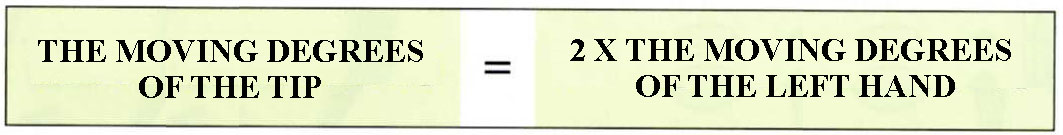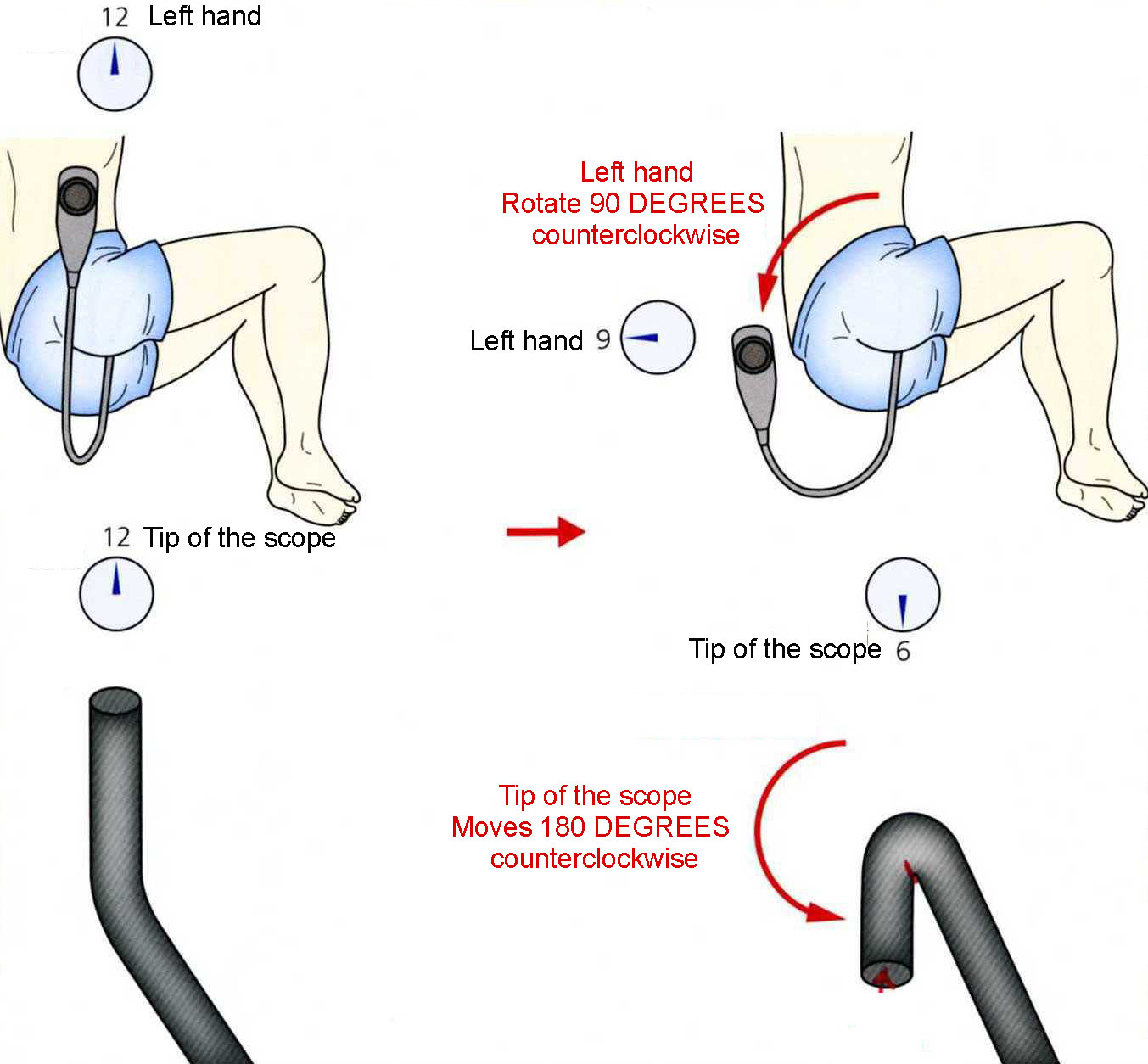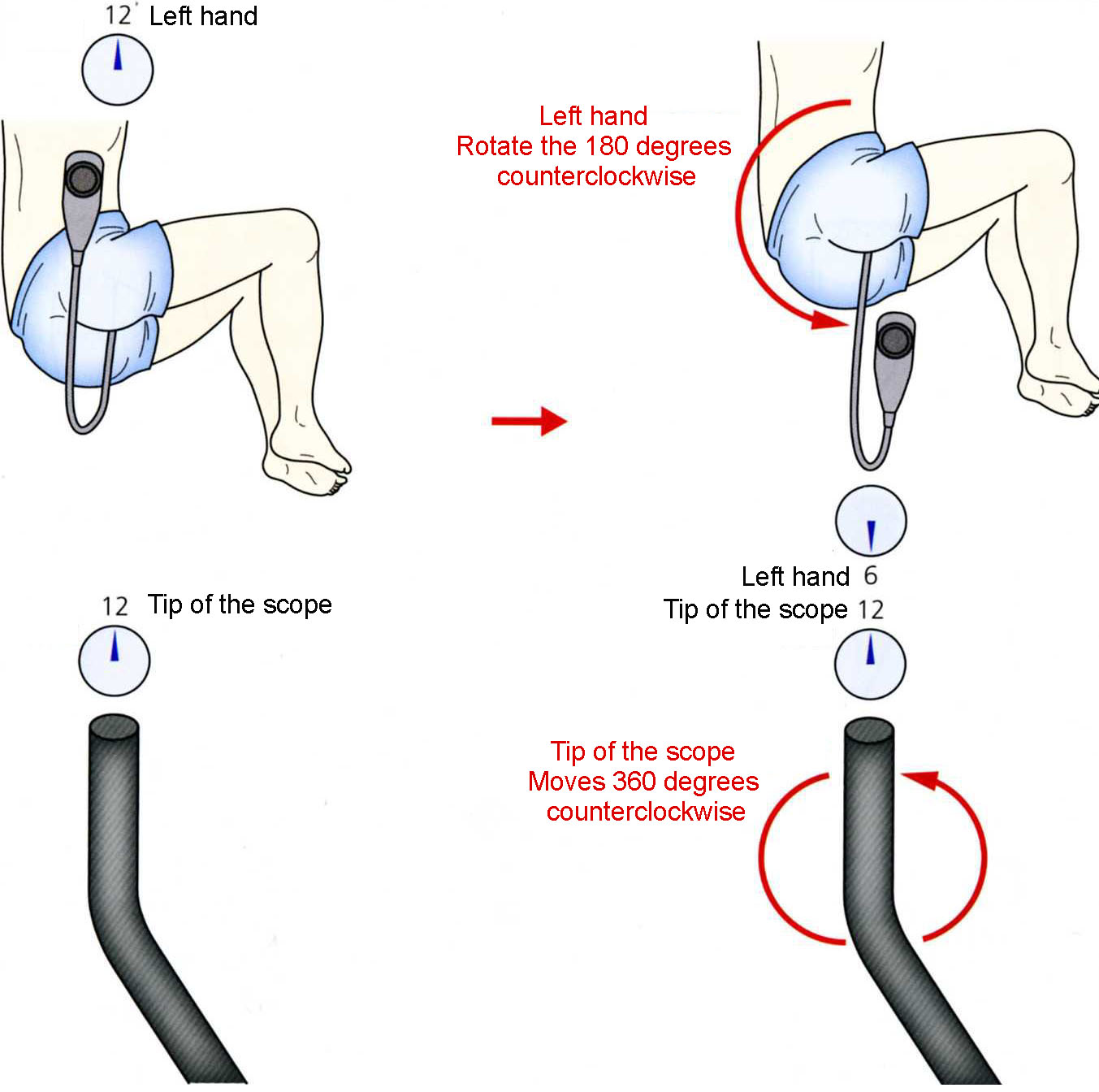11 THE RULE OF THE "DOUBLE ANGULAR VELOCITY" -IT IS POSSIBLE TO TELL THE ANGLE OF THE TIP WITHOUT SEEING.

 THE HOME POSITION OF THE SCOPE AND THE ANGLE OF THE TIP

At the home position the monitor view corresponds to the gravity direction: when the endoscopist uses the up angulation the tip of the scope would point in 12 o'clock direction as seen from the endoscopist (Chart 11-1). If not, that means:

1) The scope is not on the same plane. And/or

2) The left palm is not facing upward. When these two points are met, it is possible to tell the angle of the tip of the scope from the left hand's position. In other words, the left hand's position plays a role as the "angle sensor" of the tip.

Å@1) The scope is on the same plane. 2) The left palm is horizontal.

CHART 11-1 THE HOME POSITION OF THE SCOPE

WHEN USING THE UP ANGULATION THE TIP OF THE SCOPE POINTS UPWARD.

Å@

As the left hand makes a circle from the home position, the tip of the scope moves twice as much angle as the left hand. This is "the rule of the double angular velocity" (Chart 11-2). Based on this rule, it is possible to tell the position of the tip, which is invisible in the patient's body, with the left hand's position. There are two requirements for this rule to apply:

Å@CHART 11-2 "THE RULE OF THE DOUBLE ANGULAR VELOCITY" THE DIRECTION OF THE TIP CAN BE ASSUMED WITH THE LEFT HAND'S POSITION

Å@

EXAMPLE 1.

As you rotate the left hand 90 DEGREES counterclockwise from the home position, the tip of the scope moves 180 DEGREES counterclockwise and points downward. That is, "the left hand at 9 o'clock, the tip at 6 o'clock." (Chart 11-3)

Å@CHART 11-3 180 DEGREES COUNTERCLOCKWISE ROTATION WHEN THE LEFT HAND ROTATES 90 DEGREES COUNTERCLOCKWISE, THE TIP WOULD ROTATE 180 DEGREES COUNTERCLOCKWISE.

Å@

EXAMPLE 2.

As you rotate the left hand 180 degrees counterclockwise from the home position, the tip of the scope moves 360 degrees counterclockwise and points upward. That is, "the left hand at 6 o'clock, the tip at 12 o'clock." (Chart 11-4)

Å@CHART 11-4 360 DEGREES COUNTERCLOCKWISE ROTATION WHEN THE LEFT HAND ROTATES 180 DEGREES COUNTERCLOCKWISE, THE TIP WOULD ROTATE 360 DEGREES COUNTERCLOCKWISE.

Å@

EXAMPLE 3.

As you rotate the left hand 90 degrees clockwise from the home position, the tip of the scope moves 180 degrees clockwise and points downward. That is, "the left hand at 3 o'clock, the tip at 6 o'clock." (Chart 11-5)

Å@CHART 11-5 180 DEGREES CLOCKWISE ROTATION WHEN THE LEFT HAND ROTATES 90 DEGREES CLOCKWISE, THE TIP WOULD ROTATE 180 DEGREES COUNTERCLOCKWISE.

Å@

 MOVIE 11-1 THE RULE OF DOUBLE ANGULAR VELOCITY This rule is the basis for the Singular Method.

Å@

 MOVIE 11-2 CONFRIM THE RULE OF DOUBLE ANGULAR VELOCITY The clock in the upper right corner indicates the position of the left hand and the clock in the upper left corner indicates the direction of the tip of the scope. Q1. What degrees does the tip rotate when the left hand rotates 90 degrees counterclockwise? Q2. What degrees does the tip rotate when the left hand rotates 45 degrees counterclockwise? Q3. What time is the tip at when the left hand is at 10:30? Q4. What time is the tip at when the left hand is at 7:30? Q5. What time is the left hand at when the tip is at 9 o'clock? Q6. What time is the left hand at when the tip is at 6 o'clock? A1. 180 degrees, A2. 90 degrees, A3. 9 o'clock, A4. 3 o'clock, A5. 10:30, A6. 3 o'clock or 9 o'clock

Å@